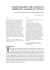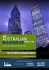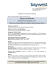# some stratified sampling plans in replicated designs

## Transcription

some stratified sampling plans in replicated designs
```Reprtnted from Estadfstica, Journal o f the Inter-American Statistical Institute,
Dtciembre de 1959
SOME STRATIFIED SAMPLING PLANS IN
REPLICATED DESIGNS
SOME STRATIFIED SAMPLING PLANS IN
REPLICATED DESIGNS
RESUMEN
El prop6sito de este articulo es describir algunos de 10s.
posibles procedimientos de muestreo estratificado, y comparar
las ganancias en la precisibn que con cada uno de ellos se
obtiene con 10s respectivos costos. Los procedimientos que el
autor presenta siguen diseiios de muestreo reiterado con aplicaci6n especial de las muestras interpenetrantes de Mahalano1)is.
La estratificacibn es una forma de usar la informacibn
estadistica disponible para lograr una estimaci6n mejor que la
que seria posible obtener de otro modo. La informaci6n
existir para todas las unidades de muestreo en el marco (como
en el 6ltimo censo), o puede exigir pruebas o entrevistas en
una muestra preliminar. La estratificaci6n tiene en realidad
muchos signilicados y muchas fornias de aplicacibn.
U n a ventaja de la eslratificaci6~1,adem8s de la ganancia
en la precisibn, es la de disrdinuir el wficiente del cuarto momento, con mejoramiento en la estimaciiln de la varianza de f
El autor describe varios planes de estratificaci61-1 que distingue con las letras A, B, C, etc., y emplea el plan A colno
elemento principal de las comparaciones entre ellos.
The purpose of stratification. MB&, h m e g i k s Hnsuses, complete
testing, or sampling, are already stra
Material, as it comes to us in the fra
natural stratification is automatic
census statistics, which are usually
Another example is industrial product, each fsem e i c h emerges at the
end of the production-line in the order in which i t \$mmade.
It sometimes pays to rearrange the sampling units into more
homogeneous groups called strata. We sometimes rearrange all the sampling
units in the frame before we draw the sample, and sometimes we rearrange
only the sampling units in the sample, depending on costs. The aim of
this paper is to describe some of the possible procedures of stratified
sampling, and to compare the gains in precision with the cost.. The
prockdures will follow replicated sampling designs as a special application
of Mahalanobis's interpenetrating samples.
* Consultant in Statistical S~trveys, 4926 Butterworth Place. Washington 16: Professor of
Stratification is one way to use statistical information in our possessiorl
to acquire a better estimate than would be possible otherwise. The statistical
information that we use in a stratified design may already exist for all
the sampling units in the frame (as from the last census), or i t may
require tests or interviews of a preliminary sample. Stratification has in
fact many meanings and many ways of application.
An advantage of stratification, aside from gain in precision, is a
decrease in the fourth moment coefficient, with improvement in the
estimate of Var Z.
1
Summary description of several p l ~ n s . 1 I shall give at this point a
brief summary of several stratified plans. I shall distinguish the various
plans by the letters A, B, C, etc. Plan A is the basis for comparison, as
it will be a replicated sample oE the material just as it comes to us in the
frame, without further stratilication. \Ye cannot be sure in the case of
new material just how effective the natural stratification is, but Plan A,
as we use it, will capture any possible gain from this source. We may
think of Plan A as a proportionate stratified sample, where the strata
are zones in the frame. Later sections show directions in more detail.
Plan A. Sample the frame as it is, xviih no rcarrangclncnt
THE PROPORT'IOXS P, KNOWN
Classify the xvl~ole fralne
Plan B . ClassiQ all the sampling units of the frame. Then use proportionate
allocation.
Plan C . Classify all the sampling units oi the I n m e . Then u x Ney~uanallocation.
Classify only the n sampling units or the salnple
Plan D . Draw the sample as in Plan A, the size being determined exactly as
SEIr Plan B. Then classify the sampling units into the various strata. Use the entire
sample; no thinning.
Plan E . Draw the sample as in Plan .I\, the size I,eing determined as in Plan C.
Then classify the sampling units into the various strata. T h i n the strata to reach the
Neyman ratios n , : n , . Choose the number n1 so that with as little thinning as possible,
the total sample will turn out to k about equal to the desired size n .
Classify only enough sampling units to fill the quotas n t .
Plan F . Fix the sample sizes n, by proportionate allocation, as in Plan B. Then
draw sampling units one by one from the zones in the frame and classify them into
the various strata until all the quotas n, are full. Discard any sampling unit that
beloags to a stratum whose quota is lull. Form the estimates and the variances as
I
in pian B.
Plnn G . Fix the sample-siles 1 1 . by Neytnar~ ;rllotation, ns in Plali C. Proceed
otherwise as in Plan F, but f o r n ~ ~ h ccstio~atcs ant1 LIIC variances as in Plan C .
T h e theory apd other illustrations of the varior~s plans appear in Chapter I 5 of my book
DICIEMBRE
1959
I'ROPORTIONS PC NOT KNOWN
Classify only a preliminary sample of size N' to estimate the proportions P , .
Plan H. Draw as in Plan A a preliminary sample of fixed size N1.Classify only
these sampling units, not the whole frame. Then thin proportionately the samples
from the various strata to reach a total specified sire. Form the estimate X as directed
later.
Plan I . Draw as in Plan A a preliminary sample of fixed size N', and classify
only the sampling units in this preliminary sample, not the whole frame. Then thin
the samples from the various strata by the use of ratios dictated by the Neyman
allocation to reach a total specified size. Form the estimate X as directed later.
STRATIFICATION BEFORE SELECTION
Some notation and definitions in the frame.
we go on, to introduce some definitions.
I t will save time, before
the average population
per sampling unit
the weighted average
standard deviation
within strata
the weighted average
variance within strata
if= PI (al - a)"
=
P2 (a2- a)'
"
+ P, (a3- a)2
P , U ? + p 2 a ; + P , U ~- a
2
the variance
between strata
the total variance
the reverse internal
variance (P, Qi = 1)
+
the average reverse
internal standard
deviation
I t is .helpful to see some of these definitions arrayed in Table 1.
More detailed description of Plan A. I now give fuller directions
for the plans, step by step, starting with Plan A. The reader will in practice
discover his own short-cuts, which will vary with local conditions and
preferences. T h e notation will be obvious, I believe, by a glance at Table 2.
1
I
I. Decide with the aid of' the equation
Var zj=
;(
1'
-T
)o2
[Plan A]
=-
n.
the size n of the sample required. Or, t:hc equivalent for111
+1
of Equation (8) may be handier, as it leads directly to Step 2. The symbol a;
is the value desired for the standard error of 5 . The symbol a2 is the
average variance between sampling units within zones. I n sampling new
material, it is usually wise to be conservative and to assume that there
will be little or no gain from the natural stratification, which means that
we should at first set IS^ equal to the predicted total variance between the
sampling units in the frame. Later on, with experience, we may decrease a2
to allow for the gain from natural stratification in the frame.
2 . Compute the zoning interval Z = 2 N / n for 2 subsamples, 10 N/n
for 10 subsamples. Form zones of' Z consecutive sampling units in the
frame.
3 . Draw with raridom numbers between 1 and 2, 1 sampling unitfrom every zone. These drawings form S.ubsample 1. Draw another sampling unit from every zone for Subsample 2, and likewise for the other
TABLE '2. NOTATIONS AND DEFINITIONS FOR THE SAMPLE
Population
in the
sample
Stratum
MeanhQpuIation
Fer
sampl~ng unit
,
Estiited
total
population
Variance
of this
estimate
q 2-population in
Stratum 1
za population in
Stratum 3
-
Sum ....................
x
X
Var X*
The variances are additive only if the N i (or P i ) are known and used in the estimate X .
subsamples if any. Mark each sampling unit to show which subsample it
belongs to, and which zone it came from.
4.. Carry out the interviews or the tests on the entire sample
5.
6.
where
Form the x-population by subsample.
Form from Subsample i the estimate.
("
is the x-population in Subsample z .
(m
E
the [email protected],whme
s(kJ,
is
on attained, For e%ample, if there were 10
ne, * we c ~ u k iuse the range between the
of the 10 vaIum of sW to form the estimate
it is advisable to tabu1;ate the results
fficient degrees of freedom, and
h the @timate. The camputation by which to
ib in my ease valid and 8imple with a ~ p l i c a t e d
We proceed now to give fuller
I3 and C that aIl the samplfng
him! been classified into strata and cotmfed, and that
in Stratum 1 have w i a l numbem 1 to Nl,in Stratum
maintain in each stratum the
PLAN B
1.
Decide with the help of the equation
[Plan B]
-. -1 J'w2
n
2 . Compute the zoning interval Z = 2 N / n for 2 subsamples, 10 N / n
for 10 subsamples. Form zones of Z sampling units in all strata.
3 . Draw with random numbers between 1 and 2, 1 sampling unit
from every zone, onward through all strata. These drawings form
Subsample 1. Draw another sampling unit from every zone for Subsample
2, and likewise for the other subsamples if any. Mark each sampling unit
to show which subsample it belongs to, and which stratum, and which
zone it came from.
4. Carry out the interviews or the tests on the entire sample.
5 . Form the X-populations by subsample: designate them x(l), ~ ( 2 1 ,
etc., where x(o is the x-population in Subsample i through all strata.
6 . Form from Subsample i estimates as in Step 6 of Plan A .
7. Form the final estimate X as in Step 7 of Plan A .
8. Estimate the precision obtained, as in Plan A . No weighting is
required, because all the sam ling unfts in the frame, regardless of stratum,
have in proportionate strati ied sampling (Plan 13) the same probability
of selection. We may, of course, form separate estimates by stratum, if
we need them. The estimates of X md of Var X are additive, as in
Table 2. The degrees oE freedom, h ~ w v e r ,are not additive.3
'
I
I
!
Neyman allocation (Plan C). ape may be able in some problems to
q rtltering n , and nz in proportion
improve on proportionate sampling l
to al and a2. This is so when it is posible to form strata so that their
variabilities (as measured by c,, a d us) are distinctly different. Such a
plan was first put into practice by N e ~ r n a n .Plan
~ C will denote Neyman
allocation when we fix the sample-sizes in advance. The steps in Plan
C follow
PLAN C
1. Decide with the aid of the equation
. (?to)"
-n
(15)
the size n of the sanlple required. Conipute for Stratunl i the sample-size.
This fmnlula in a more general form for any number of strata (or tliick zones), and for
any number of degrees of freedom in each stratum, was iirst given explicitly by F.E. Satterthwaite.
"An approximate distribution of estimates of variance components," Biometries, volume 2, 1946:
pp. 110-114.
4 J. Neyman, "On the two different aspects of the representative method,'' Jorcrnal of the
Royal Statistical Society, volume XCVTI, 1934: pages 558-606. The mathematical equations for
Neyman allocation were nevertheless published earlier by A. Tschupmw, Metron, No. 3, 1923,
page 672, but so far as I know, Tschuprow made no use of his formulas.
J
I
rm SAMPLING
PLANS
723
.Compute the zoning intervals & = 2 Ni/nc for 2 subsamples,
,for 10 subsamples. See Step 2 under Plan B. Form the zones in
ous strata with the zoning intervals just calculated; see Step 2 under
. The procedure is
to make the selections from every stratum in
Draw with random numbers between 1 and Z,, 1 sampling unit
numbers between I and 5,
2; etc. The sample so drawn
t from every zone for Subsample
any. Mark each sampIing unit
which submpIe i t belongs to, and which stratum, and which
iews or tests on the entire sample.
i the x-populations xt3, z\$),xf) stratum
(17)
s for the total 2-population
estimates from Subsample i
For
stratum, in which case the
for Stratum 1
Xz = z2z2
for Stratum 2
Etc.
for Stratum 1
for Stratum 2
DICIEMBRE
1959
for k subsamples. Varx signifies an estimate of Var x. Zl is the average
of the k individualx-populations ff) in Stratum 1, f 2 has a similar
definition in Stratum 2 . The estimates and their variances are additive,
as in Step 8 under Plan R; hence we form
Plans D, E, F, G . These plans
advance knowledge of PI, P2, PS.
(like Plans B and C) all require
PLAN D
1.
Decide with the aid of the equation
the size n of the sample required. Proceed to draw this sampIe exactly as
in Plan A .
2. Compute the zoning intervals Z = 2AT/n for 2 subsamples, 10 N / n
for 10 subsamples. Form zones of Z sampling
units in the frame.
3 . Draw with random numbers between 1 and Z, 1 sampling unit
from every zone. These drawings form Subsample 1 .
4. Classify each sampling unit into its proper stratum. Mark each
sampling unit to show that it belongs to Subsample 1, which stratum, and
which zone it came from.
5. Repeat Steps 3 and 4 for Subsample 2, and for the other subsamples if any. The sample-sizes nl(*),nz('), n,(" thus drawn will be
random variables. (The subscripts refer to the strata; the superscript is
the subsample.
.)
6 . Carry out the interviews or the tests on the entire sample.
denote as before the s-population in Subsample i from
7. Let
Stratum 1, with similar definitions for 2 \$ ) , xt) . Form for Subsample i
the estimate
-
XI")
```

### 6734-2 CS6000 Leaflet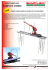### Allocation of Sample Sizes in bi-objective Stratified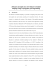### South Towne Expo Center - The Great Salt Lake Business Conference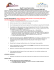### South Towne Expo Center and Salt Palace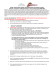### CS6000 - TekPro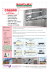### 2011 Bil Jac Sponsorship Flyer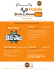### standard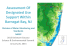### Acceptance Sampling Using Statgraphics Centurion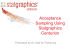### AGENCY Arc Worldwide Manila, Philippines Creative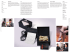### Sampling Systems for Hayabusa and follow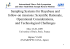### Ch 5: Integumentary System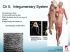### Social inequalities and transition to adulthood in contemporary Mexico